#Interactive Real Analysis

Next | Previous | Glossary | Map

## 4.2. Convergence Tests

### Abel Convergence Test

Consider the series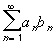. Suppose that
1. the partial sums S N =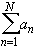form a bounded sequence
2. the sequenceis decreasing
3. lim b n = 0
Then the seriesconverges.
This test is rather sophisticated. Its main application is to prove the Alternating Series test, but one can sometimes use it for other series as well, if the more obvious tests do not work.

 Examples 4.2.18:Does the sumconverge or diverge ?Does the series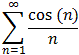converge or diverge ?
Proof:

First, we need a lemma, called the Summation by Parts Lemma:

Lemma: Summation by Parts

Consider the two sequencesand. Let S N =be the n-th partial sum. Then for any 0mn we have: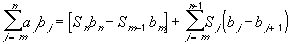Assuming this lemma is proved, we will use it as follows for Abel's Test:

First, let's assume that the partial sums S N are bounded by, say, K. Next, since the sequenceconverges to zero, we can choose an integer N such that | b n | </ 2K. Using the Summation by Parts lemma, we then have: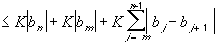But the sequenceis decreasing to zero, so in particular all terms must be positive and all absolute values inside the summation above are superfluous. But then the sum is a telescoping sum. All that remains is the first and last term, and we have: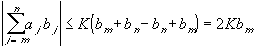But by our choice of N this is less thanif we choose n and m larger than the predetermined N. This proves Abel's Test.

What remains to do is the proof of the lemma, which can be found here.Next | Previous | Glossary | Map